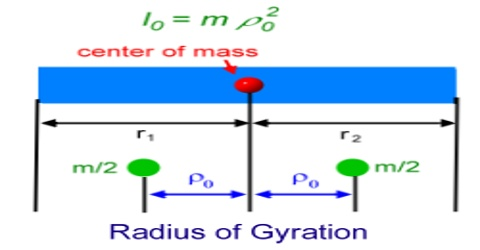The radius of gyration is used to compare how various structural shapes will behave under compression along an axis. It is used to predict buckling in a compression member or beam.

If total mass of a body is considered to be concentrated at a fixed point so that moment of inertia of that point with respect to a fixed axis is equal to the total moment of inertia of that rigid body about that axis, then we can write,

I = ∑ mr2 = MK2 … … … (1)

Here, M = ∑m = mass of the whole body and K = distance of the point from the axis of rotation where total mass of the body is concentrated. where I = second moment of area

A = area of material in the cross section

K is called radius of gyration and can be defined in the following way;

Definition: If a point of a rigid body is considered where total mass of the body is concentrated and moment of inertia at that point with respect to an axis of rotation is equal to the moment of inertia of the whole body, then the distance of that point from the axis is called radius of gyration.

From equation (1) we get, K = √(I/M)

Radius of gyration of a body with respect to a fixed axis is 0.2 m, means that if moment of inertia is determined considering that total mass of the body is concentrated at a distance 0.2 m from that axis, and then total moment of inertia is found out.